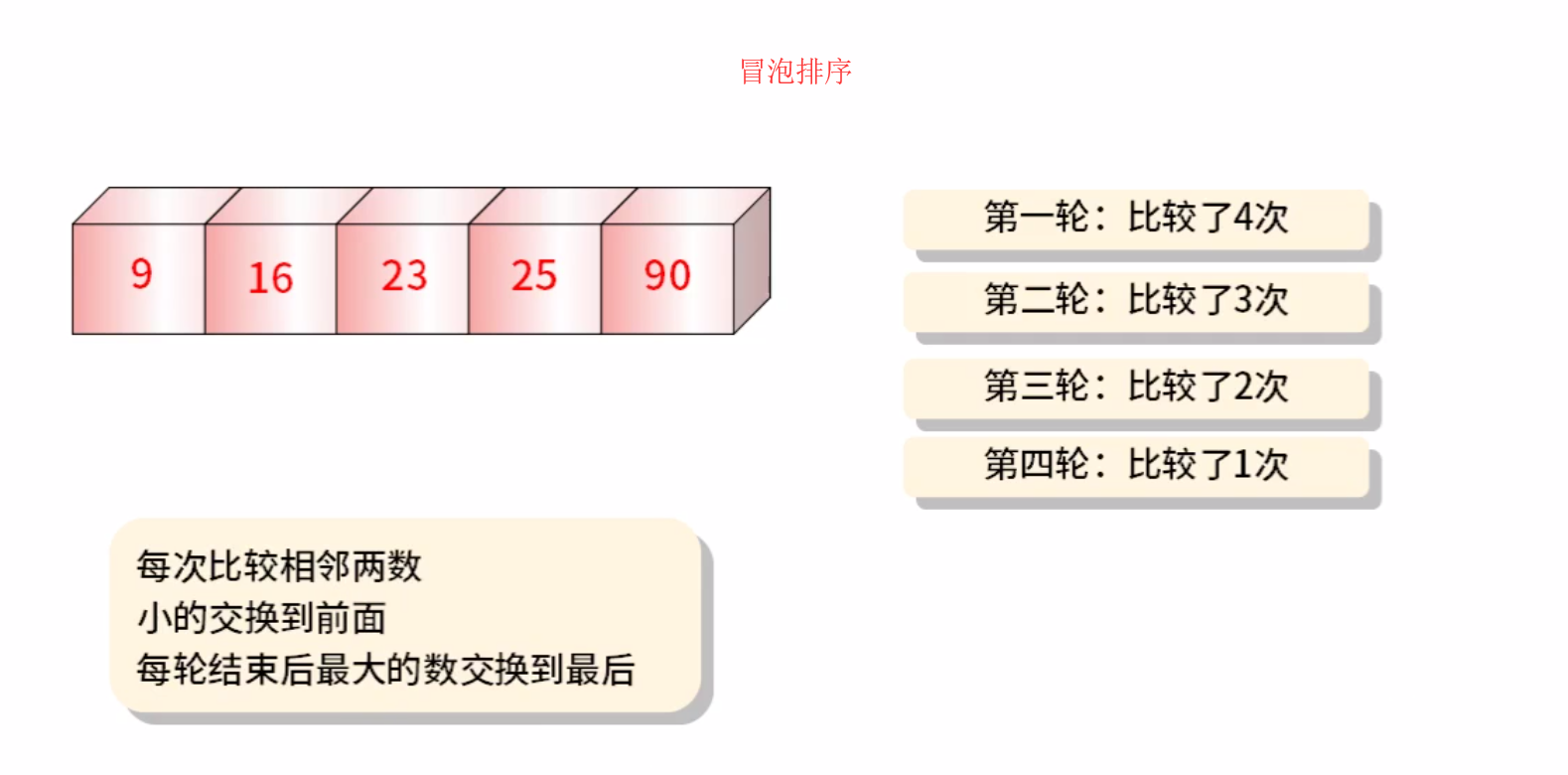# Java经典算法之冒泡排序

……``````/*冒泡排序*/
package maopao;

import java.util.Arrays;

public class maopao {

public static void main(String[] args) {
//定义个静态数组
int[] n  = {41,72,93,456,12};
//循环 控制比较轮数
//减1是因为 最后一个最大数不循环了 不比较了
for(int i=0;i<n.length-1;i++) {
//相邻的进行比较
//减i 是减去已经排好的！ 不再进行排
for(int j=0;j<n.length-1-i;j++) {
//< 从大到小排序
//> 从小到大排序
if(n[j]>n[j+1]) {
//定 中间变量进行交换位置！
int tem = n[j];
n[j]=n[j+1];
n[j+1]=tem;

}
}
}
//输出为 字符串类型！ api
System.out.println(Arrays.toString(n));

}
}``````